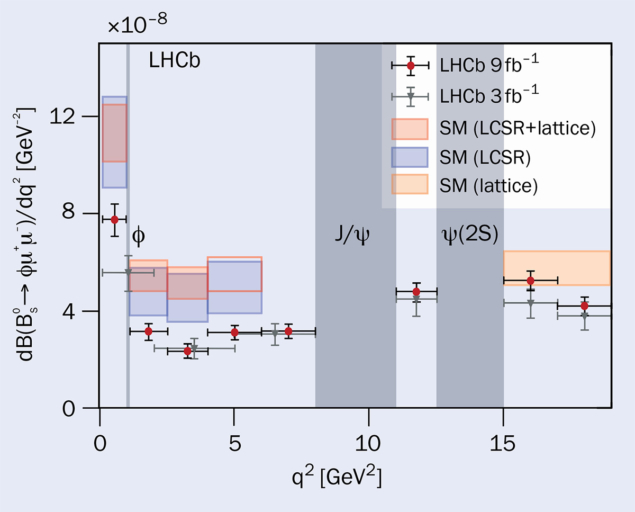# Bs decays remain anomalous

1 October 2021

A report from the LHCb experimentFig. 1. Differential branching fractions for the decay Bs → φμμ, compared with SM predictions based on light-cone sum rules (LCSR) and lattice-QCD calculations. Credit: CERN

The LHCb experiment recently presented new results on the b → sμμ decay of a Bs meson to a φ meson and a dimuon pair, reinforcing an anomaly last reported in 2015 with improved statistics and theory calculations. Such decays of b hadrons via b → s quark transitions are strongly suppressed in the Standard Model (SM) and therefore constitute sensitive probes for hypothetical new particles. In recent years, several measurements of rare semileptonic b → sℓℓ decays have shown tensions with SM predictions. Anomalies have been spotted in measurements of branching fractions, angular analyses and tests of lepton flavour universality (LFU), leading to cautious excitement that new physics might be at play.

Calculating the Standard Model prediction is more challenging than for lepton-flavour universality

At the SM@LHC conference in April, LHCb presented the most precise determination to date of the branching fraction for the decay using data collected during LHC Run 1 and Run 2 (figure 1). The branching fraction is measured as a function of the dimuon invariant mass (q2) and found to lie below the SM prediction at the level of 3.6 standard deviations in the low-q2 region. This deficit of muons is consistent with the pattern seen in LFU tests of b → sℓℓ transitions, however calculating the SM prediction for the Bs→ φμμ branching fraction is more challenging than for LFU tests as it involves the calculation of non-perturbative hadronic effects.

Calculations based on light-cone sum rules are most precise at low q2, while lattice-QCD calculations do better at high q2. A combination is expected to give the best precision over the full q2 range. If lattice-QCD calculations are not used in the comparison, increased theory errors reduce the tension to 1.8 standard deviations in the low-q2 region. The previous 2015 measurement by LHCb, which was based exclusively on Run-1 data (grey data points), was reported at the time to be approximately three standard deviations below the best theoretical predictions that were available at the time. Since then, theo­retical calculations have generally become more precise with regard to form factors, but more conservatively evaluated with regard to non-local hadronic effects.Fig. 2. The longitudinal polarisation fraction FL for the decay Bs → φμμ, compared with SM predictions based on light-cone sum rules (LCSR) and lattice-QCD calculations. Credit: CERN

### Angular information

The angular distribution of the Bs→ φμμ decay products offers complementary information. At the international FPCP conference in June, LHCb presented a measurement of the angular distribution of these decays in different q2 regions using data collected during LHC Run 1 and Run 2. Figure 2 shows the longitudinal polarisation fraction FL – one of several variables sensitive to anomalous b → sμμ couplings. The results are consistent with SM predictions at the level of two standard deviations, but may also hint at the same pattern of unexpected behaviour seen in angular analyses of other b → sμμ decays and in branching-fraction measurements.

For both analyses, LHC Run 3 will be crucial to better understanding the anomalous behaviour seen so far in Bs→ φμμ decays.